Next: Pion-Pion Lagrangian Up: Dynamical (Chiral) Symmetry Breaking Previous: Dynamical (Chiral) Symmetry Breaking

### Non-Linear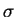Model

The non-linearmodel Gel60,Wei67 is built to describe pseudoscalar mesons of which we know that: 1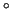they exist, 2their scalar partners don't, and 3they obey the chiral symmetry of SU(2)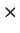SU(2). The first two facts are experimental ones, and the third one comes from the lower level (quark) theory.

The SU(2)SU(2) group is isomorphic to the O(4) group - the orthogonal group in four dimensions Gil74. Therefore, the meson fields in question can be described by four real fields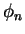,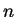=1,2,3,4, and all we need is a model for the Lagrangian density. The non-linearmodel makes the following postulate: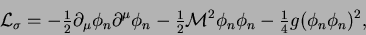(32)

where all pairs of indices imply summations. Since only lengths of vectors in the four-dimensional O(4) space appear in the Lagrangian density, it is explicitly invariant with respect to the chiral group.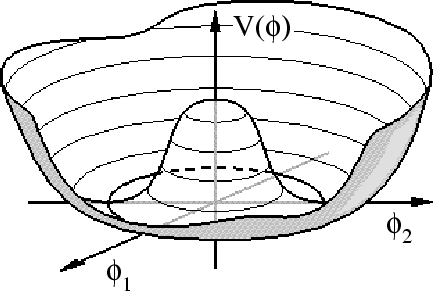The potential energy depends only on the radial variable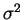=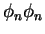, i.e.,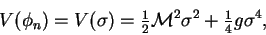(33)

but it does not depend on the orientation ofin the four-dimensional space. For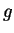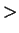0 and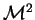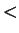0 this potential, as function of, has a maximum at=0, and a minimum at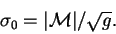(34)

However, as a function of all the four componentsit is flat in all directions perpendicular to the radial versor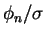. In two dimensions, such a potential is called the Mexican hat'', see Fig. 4.

Let us now consider the classical ground state corresponding to Lagrangian density (32). The lowest energy corresponds to particles at rest,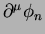=0, and resting at a lowest point of the potential energy (34). Now we have a problem - which one of the lowest points to choose, because any one such that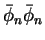=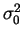is as good as any other one. However, the classical fieldsat space-time point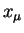must have definite values, i.e., they spontaneously pick one of the solutions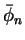out of the infinitely-many existing ones. Once one of the solutions is picked, the O(4) symmetry is broken, because the ground-state field is not any more invariant with respect to all O(4) transformations. Using the graphical representation of the Mexican hat'', Fig. 4, one can say that the system rolls down from the top of the hat, and picks one of the points within the brim.

It is now clear that fieldsdo not constitute the best variables to look at the problem, because the physics in the radial and transversal directions is different. Before proceeding any further, let us introduce variablesand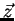that separately describe these two directions, namely,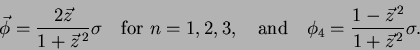(35)

Inserting expressions (35) into (32) we obtain the Lagrangian density expressed by the new fieldsand,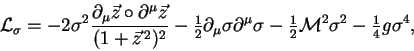(36)

where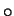'' distinguishes the scalar product in the iso-space from the scalar product in usual space, which is denoted by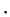''. Apart from the multiplicative factorin front of the first term, the Lagrangian density is now separated into two parts that depend on different variables. Stiffness in thedirection of the potential energy (33), calculated at the minimum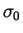, equals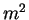=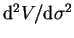=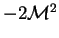0, and for large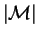is very large. Then, the fieldis confined to values very close to, and we can replace the pre-factor of the first term in Eq. (36) by. Within this approximations, fieldsandbecome independent from one another, and can be treated separately.

We disregard now the part of the Lagrangian density depending on. Indeed, the initial potential (33) has been postulated without any deep reason, and a detailed form of it is, in fact, totally unknown - it comes from the quark level that we did not at all solved. Any potential that confines the fieldto values close tois good enough. This field must remain in its ground state, because any excitations of it would bring too much energy into a meson, and again, meson's internal structure remains unresolved.Next: Pion-Pion Lagrangian Up: Dynamical (Chiral) Symmetry Breaking Previous: Dynamical (Chiral) Symmetry Breaking
Jacek Dobaczewski 2003-01-27